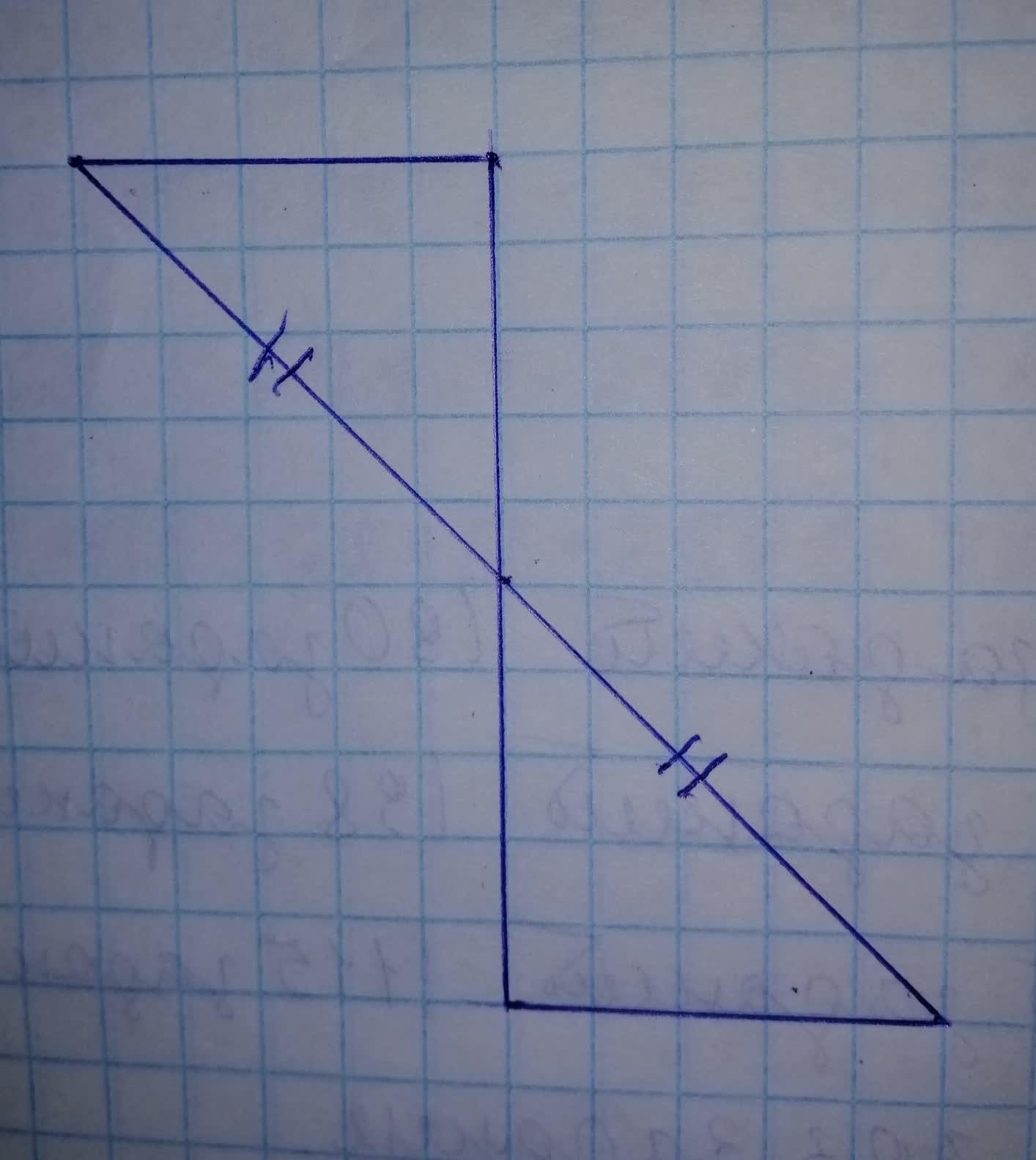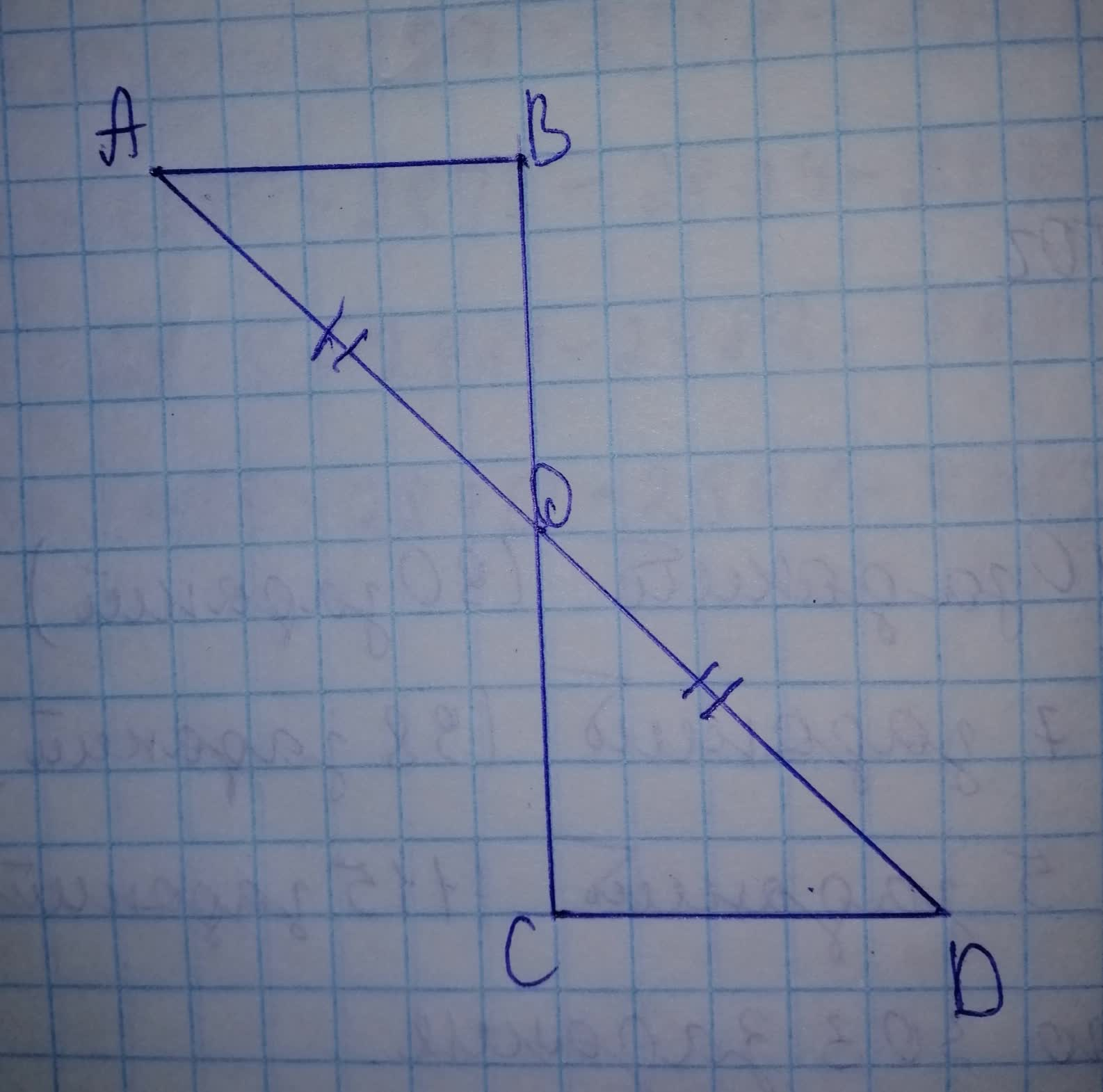# Which statement applies to these triangles: -these triangles must be congruent -tapetivk 2021-11-20 Answered
Which statement applies to these triangles: -these triangles must be congruent -these triangles might be congruent -these triangles cannot be congruent• Questions are typically answered in as fast as 30 minutes

### Plainmath recommends

• Get a detailed answer even on the hardest topics.
• Ask an expert for a step-by-step guidance to learn to do it yourself.Forneadil

Step 1Step 2
In $$\displaystyle\triangle{A}{D}{B}$$ and in $$\displaystyle\triangle{O}{C}{D}$$
$$\displaystyle{A}{O}={O}{D}$$
$$\displaystyle\angle{A}{D}{B}=\angle{C}{O}{D}$$ (vertical opposite angle.)
$$\displaystyle\triangle{A}{D}{B}\ \&\ \triangle{C}{O}{D}$$ Can or can not be congruent.